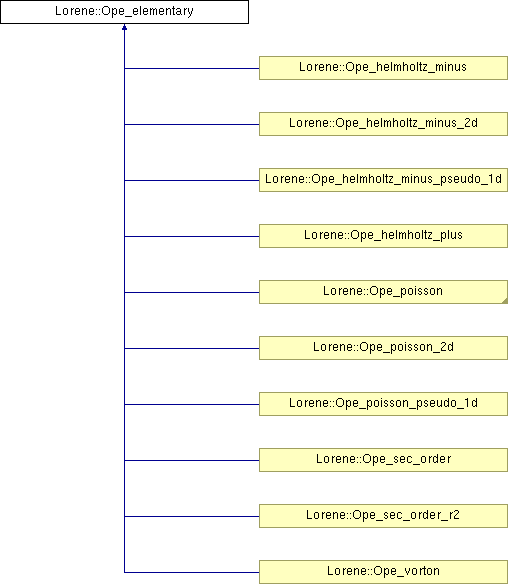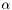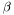# Lorene::Ope_elementary Class Reference [General PDE solvers (under development)]

Basic class for elementary elliptic operators. More...

#include <ope_elementary.h>

Inheritance diagram for Lorene::Ope_elementary:List of all members.

## Public Member Functions

virtual ~Ope_elementary ()
Destructor.
double val_sh_one_minus () const
Returns the value of the first homogeneous solution at the inner boundary.
double val_sh_one_plus () const
Returns the value of the first homogeneous solution at the outer boundary.
double der_sh_one_minus () const
Returns the value of the derivative of the first homogeneous solution at the inner boundary.
double der_sh_one_plus () const
Returns the value of the derivative of the first homogeneous solution at the outer boundary.
double val_sh_two_minus () const
Returns the value of the second homogeneous solution at the inner boundary.
double val_sh_two_plus () const
Returns the value of the second homogeneous solution at the outer boundary.
double der_sh_two_minus () const
Returns the value of the derivative of the second homogeneous solution at the inner boundary.
double der_sh_two_plus () const
Returns the value of the derivative of the second homogeneous solution at the outer boundary.
double val_sp_minus () const
Returns the value of the particular solution at the inner boundary.
double val_sp_plus () const
Returns the value of the particular solution at the outer boundary.
double der_sp_minus () const
Returns the value of the derivative particular solution at the inner boundary.
double der_sp_plus () const
Returns the value of the derivative particular solution at the outer boundary.
double get_alpha () const
Returns alpha .
double get_beta () const
Returns beta}.
int get_base_r () const
Returns base_r}.
Matrice get_ope_mat ()
Returns the matrix representation.
Matrice get_ope_cl ()
Returns the banded matrix representation.
Matrice get_non_dege ()
Returns the non degenerate matrix representation.
virtual Tbl get_solp (const Tbl &so) const =0
Computes the particular solution, given the source so .
virtual Tbl get_solh () const =0
Computes the homogeneous solutions(s).
virtual void inc_l_quant ()=0
Increases the quatum number l by one unit.

## Protected Member Functions

Ope_elementary (int nbr, int baser, double alf, double eta)
Standard constructor, protected because the class is an abstract one.
Ope_elementary (const Ope_elementary &)
Constructor by copy.

## Protected Attributes

int nr
int base_r
double alpha
Parameterof the associated mapping.
double beta
Parameterof the associated mapping.
Matriceope_mat
Pointer on the matrix representation of the operator.
Matriceope_cl
Pointer on the banded-matrix of the operator.
Matricenon_dege
Pointer on the non-degenerated matrix of the operator.
double s_one_plus
Value of the first homogeneous solution at the outer boundary.
double s_one_minus
Value of the first homogeneous solution at the inner boundary.
double ds_one_plus
Value of the derivative of the first homogeneous solution at the outer boundary.
double ds_one_minus
Value of the derivative of the first homogeneous solution at the inner boundary.
double s_two_plus
Value of the second homogeneous solution at the outer boundary.
double s_two_minus
Value of the second homogeneous solution at the inner boundary.
double ds_two_plus
Value of the derivative of the second homogeneous solution at the outer boundary.
double ds_two_minus
Value of the derivative of the second homogeneous solution at the inner boundary.
double sp_minus
Value of the particular solution at the inner boundary.
double sp_plus
Value of the particular solution at the outer boundary.
double dsp_minus
Value of the derivative of the particular solution at the inner boundary.
double dsp_plus
Value of the derivative of the particular solution at the outer boundary.

## Private Member Functions

virtual void do_ope_mat () const =0
Computes the matrix of the operator.
virtual void do_ope_cl () const =0
Computes the banded-matrix of the operator.
virtual void do_non_dege () const =0
Computes the non-degenerated matrix of the operator.

## Detailed Description

Basic class for elementary elliptic operators.

Such objects describe a type of elliptic operator, in a given domain and for a given spherical harmonics. They are called by the general elliptic solver. Ope_elementary objects know how to compute the approriate particular solution for a given source and the associated homogeneous ones.

The class Ope_elementary is an abstract one: it cannot be instanciated. Specific implementation of coordinate mappings will be performed by derived classes of Ope_elementary . ()

Definition at line 102 of file ope_elementary.h.

## Constructor & Destructor Documentation

 Lorene::Ope_elementary::Ope_elementary ( int nbr, int baser, double alf, double eta )  [explicit, protected]

Standard constructor, protected because the class is an abstract one.

Parameters:
 nbr [input] number of radial points. baser [input] radial basis of decomposition. alf [input] parameterof the mapping. bet [input] parameterof the mapping.

Definition at line 45 of file ope_elementary.C.

 Lorene::Ope_elementary::Ope_elementary ( const Ope_elementary & so )  [protected]

Constructor by copy.

Definition at line 50 of file ope_elementary.C.

References non_dege, ope_cl, and ope_mat.

 Lorene::Ope_elementary::~Ope_elementary ( )  [virtual]

Destructor.

Definition at line 65 of file ope_elementary.C.

References non_dege, ope_cl, and ope_mat.

## Member Function Documentation

 double Lorene::Ope_elementary::der_sh_one_minus ( ) const [inline]

Returns the value of the derivative of the first homogeneous solution at the inner boundary.

Definition at line 211 of file ope_elementary.h.

References ds_one_minus.

 double Lorene::Ope_elementary::der_sh_one_plus ( ) const [inline]

Returns the value of the derivative of the first homogeneous solution at the outer boundary.

Definition at line 217 of file ope_elementary.h.

References ds_one_plus.

 double Lorene::Ope_elementary::der_sh_two_minus ( ) const [inline]

Returns the value of the derivative of the second homogeneous solution at the inner boundary.

Definition at line 234 of file ope_elementary.h.

References ds_two_minus.

 double Lorene::Ope_elementary::der_sh_two_plus ( ) const [inline]

Returns the value of the derivative of the second homogeneous solution at the outer boundary.

Definition at line 240 of file ope_elementary.h.

References ds_two_plus.

 double Lorene::Ope_elementary::der_sp_minus ( ) const [inline]

Returns the value of the derivative particular solution at the inner boundary.

Definition at line 254 of file ope_elementary.h.

References dsp_minus.

 double Lorene::Ope_elementary::der_sp_plus ( ) const [inline]

Returns the value of the derivative particular solution at the outer boundary.

Definition at line 259 of file ope_elementary.h.

References dsp_plus.

 virtual void Lorene::Ope_elementary::do_non_dege ( ) const [private, pure virtual]

Computes the non-degenerated matrix of the operator.

 virtual void Lorene::Ope_elementary::do_ope_cl ( ) const [private, pure virtual]

Computes the banded-matrix of the operator.

 virtual void Lorene::Ope_elementary::do_ope_mat ( ) const [private, pure virtual]

Computes the matrix of the operator.

 double Lorene::Ope_elementary::get_alpha ( ) const [inline]

Returns alpha .

Definition at line 262 of file ope_elementary.h.

References alpha.

 int Lorene::Ope_elementary::get_base_r ( ) const [inline]

Returns base_r}.

Definition at line 268 of file ope_elementary.h.

References base_r.

 double Lorene::Ope_elementary::get_beta ( ) const [inline]

Returns beta}.

Definition at line 265 of file ope_elementary.h.

References beta.

 Matrice Lorene::Ope_elementary::get_non_dege ( )  [inline]

Returns the non degenerate matrix representation.

Definition at line 285 of file ope_elementary.h.

References do_non_dege(), and non_dege.

 Matrice Lorene::Ope_elementary::get_ope_cl ( )  [inline]

Returns the banded matrix representation.

Definition at line 278 of file ope_elementary.h.

References do_ope_cl(), and ope_cl.

 Matrice Lorene::Ope_elementary::get_ope_mat ( )  [inline]

Returns the matrix representation.

Definition at line 271 of file ope_elementary.h.

References do_ope_mat(), and ope_mat.

 virtual Tbl Lorene::Ope_elementary::get_solh ( ) const [pure virtual]

Computes the homogeneous solutions(s).

 virtual Tbl Lorene::Ope_elementary::get_solp ( const Tbl & so ) const [pure virtual]

Computes the particular solution, given the source so .

 virtual void Lorene::Ope_elementary::inc_l_quant ( )  [pure virtual]

Increases the quatum number l by one unit.

 double Lorene::Ope_elementary::val_sh_one_minus ( ) const [inline]

Returns the value of the first homogeneous solution at the inner boundary.

Definition at line 200 of file ope_elementary.h.

References s_one_minus.

 double Lorene::Ope_elementary::val_sh_one_plus ( ) const [inline]

Returns the value of the first homogeneous solution at the outer boundary.

Definition at line 205 of file ope_elementary.h.

References s_one_plus.

 double Lorene::Ope_elementary::val_sh_two_minus ( ) const [inline]

Returns the value of the second homogeneous solution at the inner boundary.

Definition at line 223 of file ope_elementary.h.

References s_two_minus.

 double Lorene::Ope_elementary::val_sh_two_plus ( ) const [inline]

Returns the value of the second homogeneous solution at the outer boundary.

Definition at line 228 of file ope_elementary.h.

References s_two_plus.

 double Lorene::Ope_elementary::val_sp_minus ( ) const [inline]

Returns the value of the particular solution at the inner boundary.

Definition at line 245 of file ope_elementary.h.

References sp_minus.

 double Lorene::Ope_elementary::val_sp_plus ( ) const [inline]

Returns the value of the particular solution at the outer boundary.

Definition at line 249 of file ope_elementary.h.

References sp_plus.

## Member Data Documentation

 double Lorene::Ope_elementary::alpha [protected]

Parameterof the associated mapping.

Definition at line 108 of file ope_elementary.h.

 int Lorene::Ope_elementary::base_r [protected]

Definition at line 107 of file ope_elementary.h.

 double Lorene::Ope_elementary::beta [protected]

Parameterof the associated mapping.

Definition at line 109 of file ope_elementary.h.

 double Lorene::Ope_elementary::ds_one_minus [mutable, protected]

Value of the derivative of the first homogeneous solution at the inner boundary.

Definition at line 141 of file ope_elementary.h.

 double Lorene::Ope_elementary::ds_one_plus [mutable, protected]

Value of the derivative of the first homogeneous solution at the outer boundary.

Definition at line 136 of file ope_elementary.h.

 double Lorene::Ope_elementary::ds_two_minus [mutable, protected]

Value of the derivative of the second homogeneous solution at the inner boundary.

Definition at line 160 of file ope_elementary.h.

 double Lorene::Ope_elementary::ds_two_plus [mutable, protected]

Value of the derivative of the second homogeneous solution at the outer boundary.

Definition at line 155 of file ope_elementary.h.

 double Lorene::Ope_elementary::dsp_minus [mutable, protected]

Value of the derivative of the particular solution at the inner boundary.

Definition at line 173 of file ope_elementary.h.

 double Lorene::Ope_elementary::dsp_plus [mutable, protected]

Value of the derivative of the particular solution at the outer boundary.

Definition at line 177 of file ope_elementary.h.

 Matrice* Lorene::Ope_elementary::non_dege [mutable, protected]

Pointer on the non-degenerated matrix of the operator.

Definition at line 122 of file ope_elementary.h.

 int Lorene::Ope_elementary::nr [protected]

Definition at line 106 of file ope_elementary.h.

 Matrice* Lorene::Ope_elementary::ope_cl [mutable, protected]

Pointer on the banded-matrix of the operator.

Definition at line 118 of file ope_elementary.h.

 Matrice* Lorene::Ope_elementary::ope_mat [mutable, protected]

Pointer on the matrix representation of the operator.

Definition at line 114 of file ope_elementary.h.

 double Lorene::Ope_elementary::s_one_minus [mutable, protected]

Value of the first homogeneous solution at the inner boundary.

Definition at line 131 of file ope_elementary.h.

 double Lorene::Ope_elementary::s_one_plus [mutable, protected]

Value of the first homogeneous solution at the outer boundary.

Definition at line 127 of file ope_elementary.h.

 double Lorene::Ope_elementary::s_two_minus [mutable, protected]

Value of the second homogeneous solution at the inner boundary.

Definition at line 150 of file ope_elementary.h.

 double Lorene::Ope_elementary::s_two_plus [mutable, protected]

Value of the second homogeneous solution at the outer boundary.

Definition at line 146 of file ope_elementary.h.

 double Lorene::Ope_elementary::sp_minus [mutable, protected]

Value of the particular solution at the inner boundary.

Definition at line 165 of file ope_elementary.h.

 double Lorene::Ope_elementary::sp_plus [mutable, protected]

Value of the particular solution at the outer boundary.

Definition at line 169 of file ope_elementary.h.

The documentation for this class was generated from the following files:

Generated on 7 Dec 2019 for LORENE by1.6.1